# Machine Learning

Sonali Arora
28 October, 2014

## Introduction

Statistical Learning plays an important role in Genomics. A few example of learning problems would be -

• Identify the risk factors(genes) for prostrate cancer based on gene expression data
• Predict the chances of breast cancer survival in a patient.
• Identify patterns of gene expression among different sub types of breast cancer

Typically, we have an outcome measurement, usually quantitative (such as gene expression) or categorical (such as breast cancer /no breast cancer), that we wish to predict based on a set of features (such as diet and clinical measurements). We have a training set of data, in which we observe the outcome and feature measurements for a set of objects (such as people). With this data we build a prediction model, or a learner, which will enable us to predict the outcome for new unseen objects. A good learner is one that accurately predicts such an outcome. This is called supervised learning because the outcome variable guides the learning process. In the unsupervised learning problem, have no measurements of the outcome and observe only the features. Our task is to describe how the data are organized or clustered.

## Machine Learning with Bioconductor : Resources Overview

Bioconductor houses a lot of R packages which provide machine learning tools for different kinds of Genomic Data analysis. It has had many releases since its inception. The latest cutting edge development happens in the “devel” version. One can find R packages specially catered to Machine Learning for Genomic Analysis by visiting the following link and using biocViews.

For example, one can type “Clustering” in the “Autocomplete biocViews search” box and find that there are 89 packages in Bioconductor which provide “Clustering” functionality for Genomic Data. On the same page, under “StatisticalMethod” one can find R packages for other machine learning techniques -

technique packages
1 Bayesian 15
2 Classification 64
3 Clustering 89
4 DecisionTree 7
5 NeuralNetwork 1
6 SupportVectorMachines 1
7 DimensionReduction 2
8 HiddenMarkovModel 4
9 Regression 7
10 PrincipalComponent 4

## Data for this Lab

We will use two data sets in this lab - one for supervised learning and one for unsupervised learning.

For Unsupervised learning, we will use the NCI60 cancer cell microarray data which contains 6830 gene expression measurements of 64 cancer cell lines. We dropped the sub types which contain only 1 sample ( there were about 6 of them) and created a SummarizedExperiment object.

The SummarizedExperiment class is a matrix-like container where rows represent ranges of interest (as a GRanges or GRangesList-class) and columns represent samples (with sample data summarized as a DataFrame-class). A SummarizedExperiment contains one or more assays, each represented by a matrix-like object of numeric or other mode.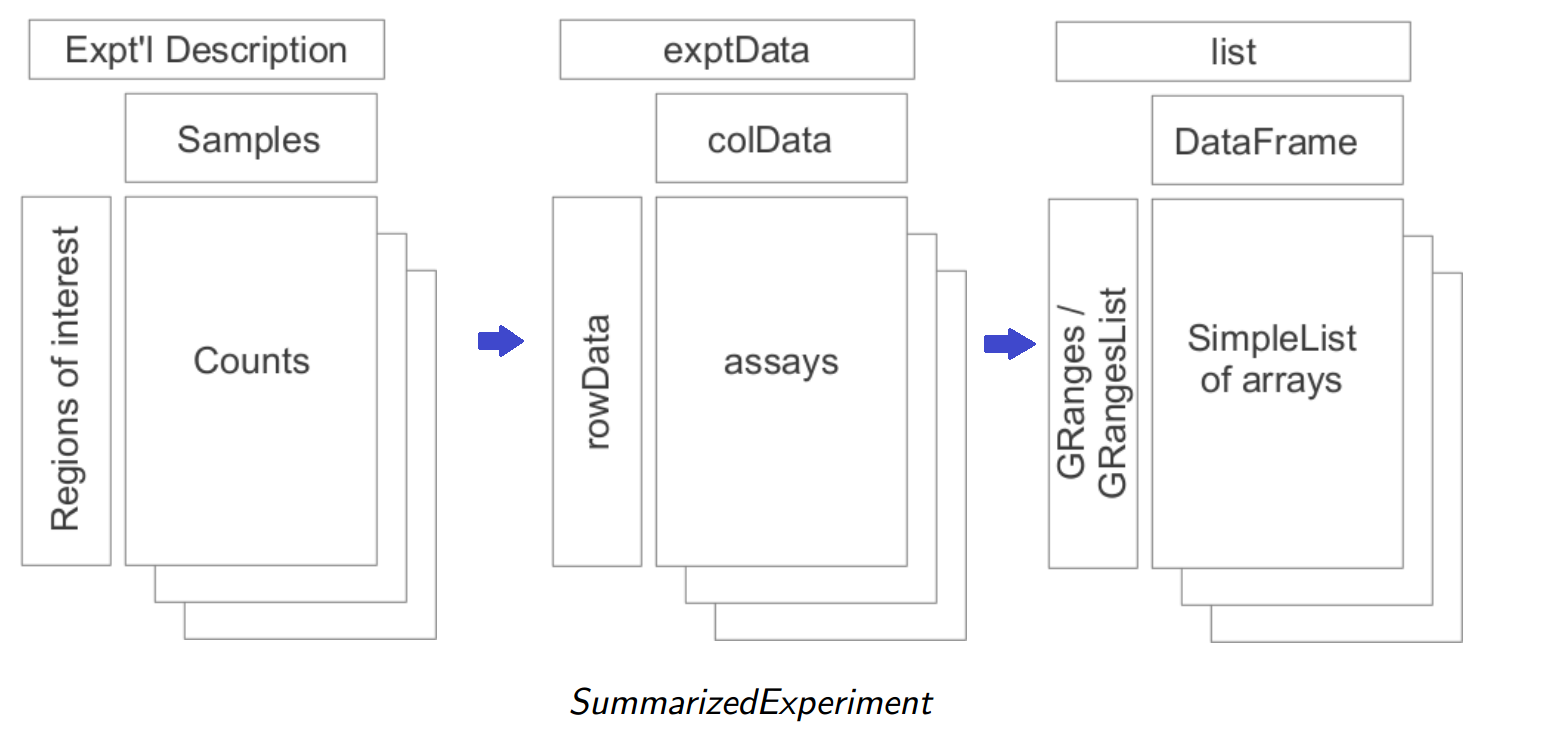This object has been made available to you, you can simply read it in using

library(GenomicRanges)
sefile <- system.file("extdata", "NCI60.Rda", package="LearnBioconductor")
nci60data <- t(assay(NCI60))
ncilabels <- colData(NCI60)


The gene expression data is stored in “assay” whereas the labels are stored in colData.

For Supervised learning, we will use cervical count data from the Biocoductor package, MLSeq. This data set contains expressions of 714 miRNA's of human samples. There are 29 tumor and 29 non-tumor cervical samples. For learning purposes, we can treat these as two separate groups and run various classification algorithms.

library(MLSeq)
filepath = system.file("extdata/cervical.txt", package = "MLSeq")


### Exercise

Download the NCI60 data from the given url and create a SummarizedExperiment object filtering out subtypes which contain only 1 sample.

## Unsupervised Learning

Unsupervised Learning is a set of statistical tools intended for the setting in which we have only a set of features $$X_1$$, $$X_2$$, ….,$$X_p$$ measured on 'n' observations. We are primarily interested in discovering interesting things on the measurement $$X_1$$, $$X_2$$, ….,$$X_p$$

Unsupervised Learning is often performed as a part of Exploratory Data Analysis. These tools help us to get a good idea about the data set. Unlike a supervised learning problem, where we can use prediction to gain some confidence about our learning algorithm, there is no way to check our model. The learning algorithm is thus, aptly named “unsupervised”.

Nevertheless, it offers great insights for our given problem. For Example, in case of our NCI60 data set, we may want to look for subgroups among our cancer samples or among genes to better understand how the disease works.

### PCA

One of the classic things that we are told as soon as we get our hands on is to make a scatter plot and visualize the data. With big data, this is not always very easy to do. For example, in our NCI60 data set we would have about ( 6830 C 2) = 6830(6830-1)/2= 23321035 scatter plots ! It is certainly not possible to look at all of them and since they each contain only a small amount of the total information present in the data set, none of them will be informative. Thus, we need a low dimensional representation of data which captures as much variation as possible.

Principal components allow us to summarize the data with a smaller number of variables that explain the variability in the data set. The process by which these principal components are computed and the usage of these components in understanding the data is known as Principal Componenet Analysis.

Let us use PCA to visualize the NCI60 data set. We first perform PCA on the data after scaling the variables (genes) to have standard deviation one and plot the first few principal components in order to visualize the data. As you can see from the following figure, with big data, biplot() is not very informative.

pcaRes <- prcomp(nci60data, scale=TRUE)
biplot(pcaRes)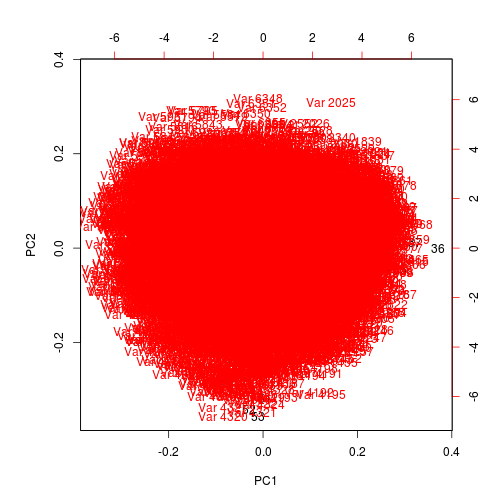So lets look at the first 3 components and see if we can gain some interesting insights from them.

# make colors as factors.
labs <- as.character(unlist(as.list(ncilabels)))

cellColor <- function(vec)
{
uvec <- unique(vec)
cols = rainbow(length(uvec))
colvec <- cols[as.numeric(as.factor(vec))]
list(colvec=colvec, cols=cols, labels= uvec)
}

par(mfrow=c(1,2))

colres <- cellColor(labs)

plot(pcaRes$x[,1:2],col=colres$colvec, xlab = "z1", ylab="z2", pch=19)
legend("bottomright", legend = colres$labels, text.col = colres$cols,
bty="n", cex=0.80)
plot(pcaRes$x[,c(1,3)], col=colres$colvec, xlab="z1", ylab="z3", pch=19)
legend("topright",  legend = colres$labels,text.col = colres$cols,
bty ="n" , cex=0.80)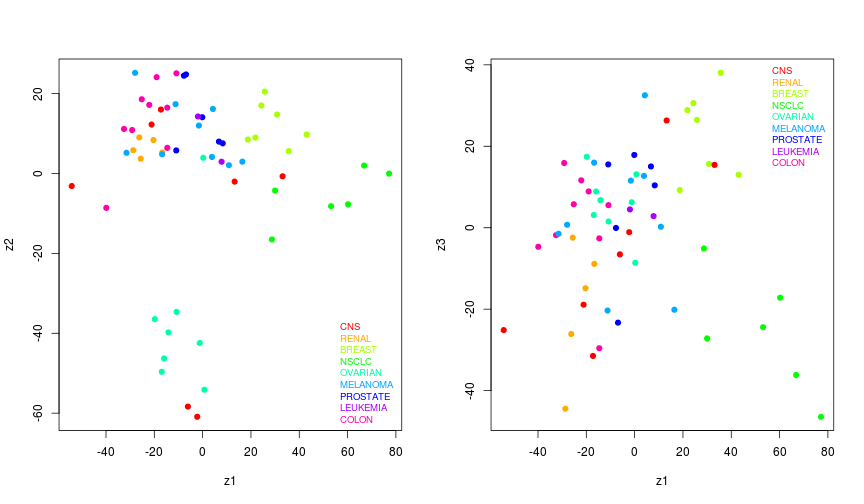par(mfrow=c(1,1))


Overall, we can conclude that the cell lines corresponding to a single cancer type tend to have similar values on the first few principal component score vectors. This indicates that cell lines from the same cancer type tend to have similar gene expression levels.

### Clustering observations

In hierarchical clustering, we start by defining some dissimilarity measure between each pair of observations (like Euclidean distance). We start at the bottom of the dendrogram, each of the n observations are considered as a cluster, the two clusters that are most similar to each other are fused together so now we have n-1 clusters. Next the two clusters that are similar together are fused so that there are n-2 clusters. This algorithm proceeds iteractively until all samples belong to one single cluster and the dendrogram is complete.

We define the dissimilarity between two clusters or two groups of observations using Linkage. There are four common types of linkage - “average”, “complete”, “single”, “centroid”. Assuming we have two clusters A and B, then -

1. Complete refers to recording the largest of all pairwise dissimilarities between observations in cluster A and observations in Cluster B.
2. Single refers to recording the smallest of all pairwise dissimilarities between observations in cluster A and observations in Cluster B.
3. Single refers to recording the average of all pairwise dissimilarities between observations in cluster A and observations in Cluster B.
4. Centroid refers to the dissimilarity between the centroid for cluster A and the centroid for cluster B.

Usually, the dissimilarity measure is the Euclidean Distance.

Lets cluster the observations using complete linkage with Euclidean distance as the dissimilarity measure in “complete linkage”.

library(dendextend)

sdata <- scale(nci60data)
d <- dist(sdata)
labs <- as.character(unlist(as.list(ncilabels)))
comp_clust <- hclust(d)
dend <- as.dendrogram(comp_clust)
leaves <- labs[order.dendrogram(dend)]
labels_colors(dend, labels=TRUE) <- cellColor(leaves)$colvec labels(dend) <- leaves plot(dend, main ="Clustering using Complete Linkage")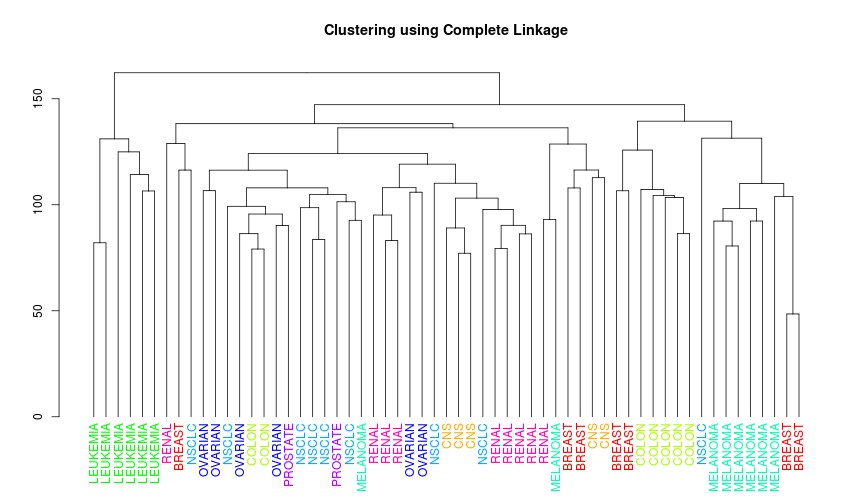### Exercise 1. Perform hierarchical clustering using average and single linkage. 2. Interpret the difference in the dendrograms. 3. Can you observe some patterns from these dendrograms? (hint: use cutree) Solutions: 1. The plots can be made with the following code - plot(hclust(d, method="average"), labels= labs, main ="Clustering using Average Linkage" , xlab="", ylab="" )plot(hclust(d, method="single"), labels= labs, main ="Clusteringg using Single Linkage" , xlab="", ylab="" )1. Briefly, complete linkage provides maximal inter cluster dissimilarity, single linkage results in minimal inter cluster dissimilarity and average results in mean inter cluster dissimilarity. We see that the results are affected by the choice of the linkage. Single linkage tend to yield trailing clusters while complete and average linkage leads to more balanced and attractive clusters. 2. For our example, we see that the cell lines within a single cancer cell type do not cluster together. But if we consider complete linkage and cut the tree at height=4 ( we tried different heights to observe patterns) we observe some clear patterns like the leukemia cell lines fall in cluster 2 and the breast cancer cell lines spread out. hc <- cutree(comp_clust, 4) table(hc, labs)  ## labs ## hc BREAST CNS COLON LEUKEMIA MELANOMA NSCLC OVARIAN PROSTATE RENAL ## 1 3 5 2 0 2 8 6 2 9 ## 2 0 0 0 6 0 0 0 0 0 ## 3 2 0 5 0 0 0 0 0 0 ## 4 2 0 0 0 6 1 0 0 0  ## Supervised Learning In supervised learning, along with the features $$X_1$$, $$X_2$$, ….,$$X_p$$, we also have the a response Y measured on the same n observations. The goal is then to predict Y using $$X_1$$, $$X_2$$, ….,$$X_p$$ for new observations. ### A simple example using knn For the cervical data, we know that the first 29 are non-Tumor samples whereas the last 29 are Tumor samples. We will code these as 0 and 1 respectively. class = data.frame(condition = factor(rep(c(0, 1), c(29, 29))))  Lets look at one of the most basic supervised learning techniques k-Nearest Neighbor and see what all goes into building a simple model with it. For the sake of simplicity, we will use only 2 predictors (so that we can represent the data in 2 dimensional space) data <- t(cervical) data <- data[,1:2] df <- cbind(data, class) colnames(df) <- c("x1","x2","y") rownames(df) <- NULL head(df)  ## x1 x2 y ## 1 865 3 0 ## 2 810 12 0 ## 3 5505 30 0 ## 4 6692 73 0 ## 5 1456 6 0 ## 6 588 2 0  This is how the data looks - plot(df[,"x1"], df[,"x2"], xlab="x1", ylab="x2", main="data representation for knn", col=ifelse(as.character(df[,"y"])==1, "red","blue"))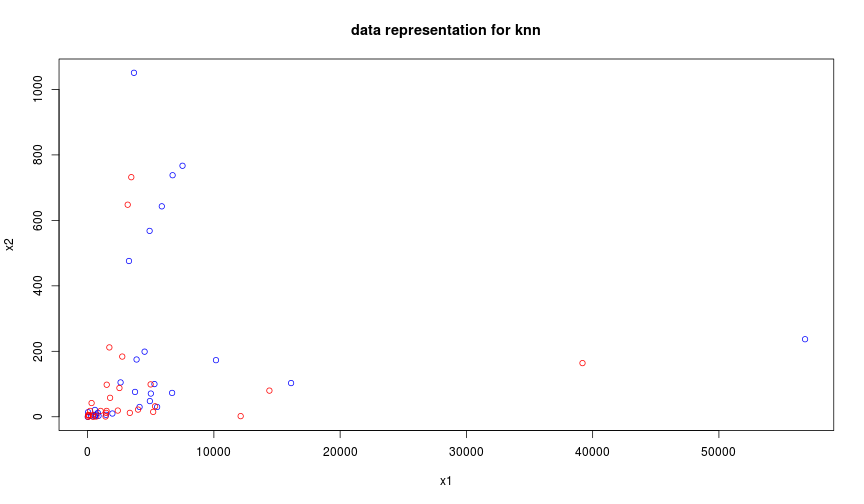Given a observation $$x_0$$ and a positive integer, K, the KNN classifier first identifies K points in the training data that are closest to $$x_0$$, represented by $$N_0$$. It estimates the conditional probability for class j as a fraction of $$N_0$$ and applies Bayes rule to classify the test observation to the class with the largest probability. More concretely, if k=3 and there are 2 observation belonging to class 1 and 1 observation belonging to class 2, then we the test observation is assigned to class1.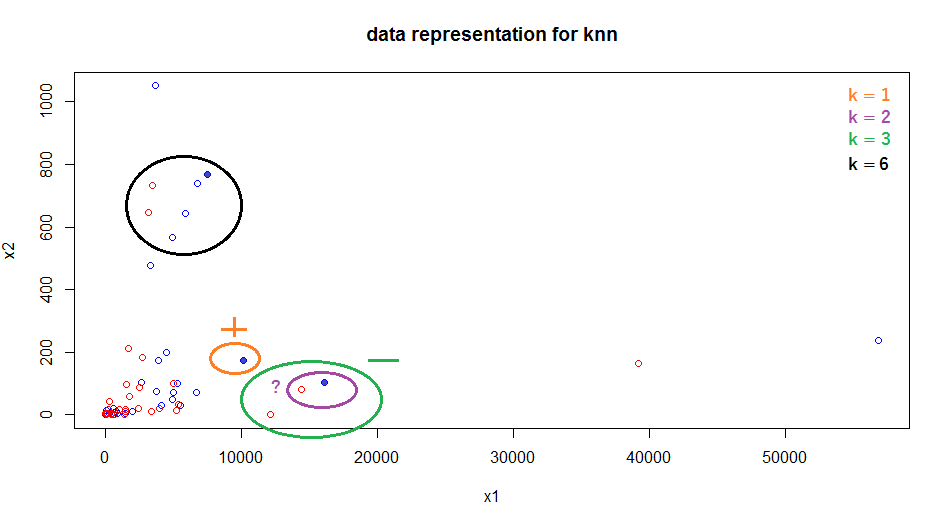For all supervised experiments its a good idea to hold out some data as Training Data and build a model with this data. We can then test the built model using the left over data (Test Data) to gain confidence in our model. We will randomly sample 30% of our data and use that as a test set. The remaining 70% of the data will be used as training data set.seed(9) nTest = ceiling(ncol(cervical) * 0.2) ind = sample(ncol(cervical), nTest, FALSE) cervical.train = cervical[, -ind] cervical.train = as.matrix(cervical.train + 1) classtr = data.frame(condition = class[-ind, ]) cervical.test = cervical[, ind] cervical.test = as.matrix(cervical.test + 1) classts = data.frame(condition = class[ind, ])  Training set error is the proportion of mistakes made if we apply our model to the training data and Test set error is the proportion of mistakes made when we apply our model to test data. For different neighbors , let us calculate the training error and test error using KNN. library(class) newknn <- function( testset, trainset, testclass, trainclass, k) { pred.train <- knn.cv(trainset, trainclass, k=k) pred.test <- knn(trainset, testset, trainclass, k=k) test_fit <- length(which(mapply(identical, as.character(pred.test), testclass)==FALSE))/length(testclass) train_fit <- length(which(mapply(identical, as.character(pred.train), trainclass)==FALSE))/length(trainclass) c(train_fit=train_fit, test_fit= test_fit) } trainset <- t(cervical.train) testset <- t(cervical.test) testclass <- t(classts) trainclass <- t(classtr) klist <- 1:15 ans <- lapply(klist, function(x) newknn(testset, trainset, testclass, trainclass,k =x)) resdf <- t(as.data.frame(ans)) rownames(resdf) <- NULL plot(klist, resdf[,"train_fit"], col="blue", type="b",ylim=c(range(resdf)), main="k Nearest Neighbors for Cervical Data", xlab="No of neighbors", ylab ="Training and Test Error") points(klist, resdf[,"test_fit"], col="red", type="b") legend("bottomright", legend=c("Training error","Test error"), text.col=c("blue","red"), bty="n")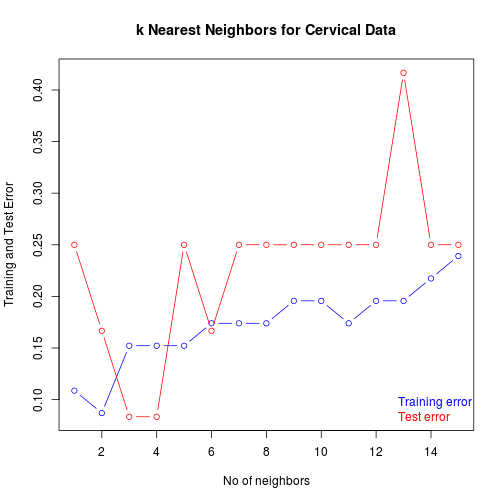Another important concept in machine learning is cross validation. Since samples are often scarse, it is often not possible to set aside a validation set ans then use it to assess the performance of our prediction model. So we use cross validation to train a better model. We start by dividing the training data randomly into n equal parts. The learning method is fit to n-1 parts of the data, and the prediction error is computed on the remaining part. This is done in turn for each 1/n parts of the data, and finally the n prediction error estimates are averaged. For example, K-fold cross validation where K=5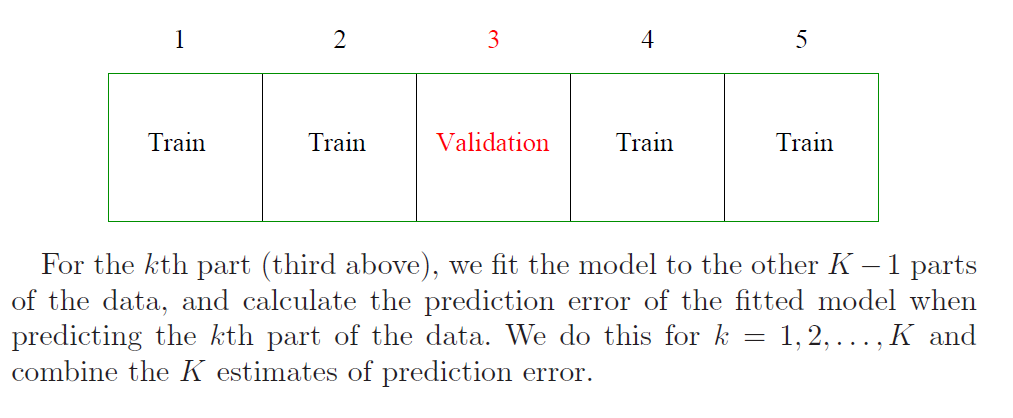As you can see, computation for this very simple learner can become quite complicated. ### Fast classification using Bioconductor. MLSeq aims to make computation less complicated for a user and allows one to learn a model using various classifier's with one single function. The main function of this package is classify which requires data in the form of a DESeqDataSet instance. The DESeqDataSet is a subclass of SummarizedExperiment, used to store the input values, intermediate calculations and results of an analysis of differential expression. So lets create DESeqDataSet object for both the training and test set, and run DESeq on it. cervical.trainS4 = DESeqDataSetFromMatrix(countData = cervical.train, colData = classtr, formula(~condition))  ## converting counts to integer mode  cervical.trainS4 = DESeq(cervical.trainS4, fitType = "local")  ## estimating size factors ## estimating dispersions ## gene-wise dispersion estimates ## mean-dispersion relationship ## final dispersion estimates ## fitting model and testing ## -- replacing outliers and refitting for 76 genes ## -- DESeq argument 'minReplicatesForReplace' = 7 ## -- original counts are preserved in counts(dds) ## estimating dispersions ## fitting model and testing  cervical.testS4 = DESeqDataSetFromMatrix(countData = cervical.test, colData = classts, formula(~condition))  ## converting counts to integer mode  cervical.testS4 = DESeq(cervical.testS4, fitType = "local")  ## estimating size factors ## estimating dispersions ## gene-wise dispersion estimates ## mean-dispersion relationship ## final dispersion estimates ## fitting model and testing ## -- replacing outliers and refitting for 60 genes ## -- DESeq argument 'minReplicatesForReplace' = 7 ## -- original counts are preserved in counts(dds) ## estimating dispersions ## fitting model and testing  Classify using Support Vector Machines. svm = classify(data = cervical.trainS4, method = "svm", normalize = "deseq", deseqTransform = "vst", cv = 5, rpt = 3, ref = "1")  ## found already estimated dispersions, replacing these ## gene-wise dispersion estimates ## mean-dispersion relationship ## found already estimated fitted dispersions, removing these ## final dispersion estimates ## Loading required package: kernlab ## ## Attaching package: 'kernlab' ## ## The following object is masked from 'package:Biostrings': ## ## type  svm  ## ## An object of class MLSeq ## ## Method : svm ## ## Accuracy(%) : 97.83 ## Sensitivity(%) : 100 ## Specificity(%) : 95 ## ## Reference Class : 1  It returns an object of class 'MLseq' and we observe that it successfully fitted a model with 97.8% accuracy. We can access the slots of this S4 object by getSlots("MLSeq")  ## method deseqTransform normalization confusionMat ## "character" "character" "character" "confusionMatrix" ## trained ref ## "train" "character"  And also, ask about the model trained. trained(svm)  ## Support Vector Machines with Radial Basis Function Kernel ## ## 46 samples ## 714 predictors ## 2 classes: '1', '0' ## ## No pre-processing ## Resampling: Cross-Validated (5 fold, repeated 3 times) ## ## Summary of sample sizes: 36, 37, 37, 37, 37, 37, ... ## ## Resampling results across tuning parameters: ## ## C Accuracy Kappa Accuracy SD Kappa SD ## 0.25 0.587 0.0541 0.0451 0.112 ## 0.50 0.804 0.5763 0.1106 0.244 ## 1.00 0.870 0.7199 0.1181 0.255 ## ## Tuning parameter 'sigma' was held constant at a value of 0.0009560732 ## Accuracy was used to select the optimal model using the largest value. ## The final values used for the model were sigma = 0.0009560732 and C = 1.  We can predict the class labels of our test data using “predict” pred.svm = predictClassify(svm, cervical.testS4)  ## found already estimated dispersions, replacing these ## gene-wise dispersion estimates ## mean-dispersion relationship ## found already estimated fitted dispersions, removing these ## final dispersion estimates  table(pred.svm, relevel(cervical.testS4$condition, 2))

##
## pred.svm 1 0
##        1 3 1
##        0 0 8


The other classification methods available are 'randomforest', 'cart' and 'bagsvm'.

### Exercise:

Train the same training data and test data using randomForest.

Solutions:

rf = classify(data = cervical.trainS4, method = "randomforest",
normalize = "deseq", deseqTransform = "vst", cv = 5, rpt = 3, ref = "1")

## found already estimated dispersions, replacing these
## gene-wise dispersion estimates
## mean-dispersion relationship
## found already estimated fitted dispersions, removing these
## final dispersion estimates

trained(rf)

## Random Forest
##
##  46 samples
## 714 predictors
##   2 classes: '1', '0'
##
## No pre-processing
## Resampling: Cross-Validated (5 fold, repeated 3 times)
##
## Summary of sample sizes: 37, 37, 36, 37, 37, 36, ...
##
## Resampling results across tuning parameters:
##
##   mtry  Accuracy  Kappa  Accuracy SD  Kappa SD
##     2   0.822     0.628  0.172        0.360
##    37   0.867     0.725  0.113        0.236
##   713   0.860     0.712  0.107        0.222
##
## Accuracy was used to select the optimal model using  the largest value.
## The final value used for the model was mtry = 37.

pred.rf = predictClassify(rf, cervical.testS4)

## found already estimated dispersions, replacing these
## gene-wise dispersion estimates
## mean-dispersion relationship
## found already estimated fitted dispersions, removing these
## final dispersion estimates

table(pred.rf, relevel(cervical.testS4\$condition, 2))

##
## pred.rf 1 0
##       1 3 1
##       0 0 8


## SessionInfo

sessionInfo()

## R version 3.1.1 (2014-07-10)
## Platform: x86_64-unknown-linux-gnu (64-bit)
##
## locale:
##   LC_CTYPE=en_US.UTF-8       LC_NUMERIC=C
##   LC_TIME=en_US.UTF-8        LC_COLLATE=C
##   LC_MONETARY=en_US.UTF-8    LC_MESSAGES=en_US.UTF-8
##   LC_PAPER=en_US.UTF-8       LC_NAME=C
##  LC_MEASUREMENT=en_US.UTF-8 LC_IDENTIFICATION=C
##
## attached base packages:
##   grid      stats4    parallel  stats     graphics  grDevices utils
##   datasets  methods   base
##
## other attached packages:
##   kernlab_0.9-19
##   class_7.3-11
##   dendextend_0.17.5
##   MLSeq_1.2.0
##   edgeR_3.8.2
##   randomForest_4.6-10
##   limma_3.22.1
##   caret_6.0-35
##   lattice_0.20-29
##  xtable_1.7-4
##  DNAcopy_1.40.0
##  cn.mops_1.12.0
##  fission_0.99.4
##  sva_3.12.0
##  mgcv_1.8-3
##  nlme_3.1-118
##  Gviz_1.10.1
##  ggplot2_1.0.0
##  PoiClaClu_1.0.2
##  RColorBrewer_1.0-5
##  gplots_2.14.2
##  DESeq2_1.6.1
##  Rcpp_0.11.3
##  org.Hs.eg.db_3.0.0
##  RSQLite_1.0.0
##  DBI_0.3.1
##  BiocParallel_1.0.0
##  VariantAnnotation_1.12.2
##  RNAseqData.HNRNPC.bam.chr14_0.3.2
##  GenomicAlignments_1.2.0
##  Rsamtools_1.18.1
##  genefilter_1.48.1
##  BSgenome.Hsapiens.UCSC.hg19_1.4.0
##  BSgenome_1.34.0
##  rtracklayer_1.26.1
##  airway_0.99.5
##  ALL_1.7.1
##  TxDb.Hsapiens.UCSC.hg19.knownGene_3.0.0
##  GenomicFeatures_1.18.1
##  AnnotationDbi_1.28.0
##  Biobase_2.26.0
##  GenomicRanges_1.18.1
##  GenomeInfoDb_1.2.2
##  Biostrings_2.34.0
##  XVector_0.6.0
##  IRanges_2.0.0
##  S4Vectors_0.4.0
##  BiocGenerics_0.12.0
##  BiocStyle_1.4.1
##  LearnBioconductor_0.1.6
##
## loaded via a namespace (and not attached):
##   BBmisc_1.7           BatchJobs_1.4        BiocInstaller_1.16.0
##   KernSmooth_2.23-13   MASS_7.3-35          Matrix_1.1-4
##  R.methodsS3_1.6.1    RCurl_1.95-4.3       XML_3.98-1.1
##  acepack_1.3-3.3      annotate_1.44.0      base64enc_0.1-2
##  biomaRt_2.22.0       biovizBase_1.14.0    bitops_1.0-6
##  brew_1.0-6           brglm_0.5-9          caTools_1.17.1
##  car_2.0-21           checkmate_1.5.0      cluster_1.15.3
##  codetools_0.2-9      colorspace_1.2-4     compiler_3.1.1
##  dichromat_2.0-0      digest_0.6.4         e1071_1.6-4
##  evaluate_0.5.5       fail_1.2             foreach_1.4.2
##  foreign_0.8-61       formatR_1.0          gdata_2.13.3
##  geneplotter_1.44.0   gtable_0.1.2         gtools_3.4.1
##  hwriter_1.3.2        iterators_1.0.7      knitr_1.7
##  labeling_0.3         latticeExtra_0.6-26  lme4_1.1-7
##  locfit_1.5-9.1       magrittr_1.0.1       markdown_0.7.4
##  matrixStats_0.10.3   mime_0.2             minqa_1.2.4
##  munsell_0.4.2        nloptr_1.0.4         nnet_7.3-8
##  plyr_1.8.1           proto_0.3-10         reshape2_1.4
##  rpart_4.1-8          scales_0.2.4         sendmailR_1.2-1
##  splines_3.1.1        stringr_0.6.2        survival_2.37-7
##  tools_3.1.1          whisker_0.3-2        zlibbioc_1.12.0